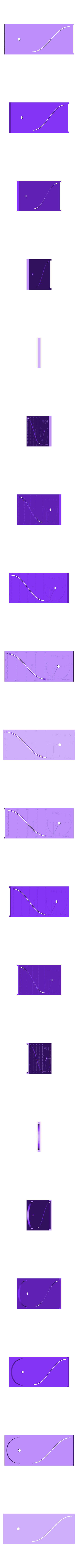# Unit Vec A Dot B = Cos Theta## 3D model description

So math was one of my favourite subjects at school thanks to some truly wonderful teachers. However I always found the way I was taught trigonometry was rather obtuse. This device can be used with students who are familiar with some basic concepts from geometry and algebra to visualise how the cosine values used in trigonometry can be computed using a unit vector dot product. The interesting thing is that when one of the vectors is [1,0] the cosine is simply the x value.

Here is a quick youtube video that explains it...

In the example provided we have an angle of 60° which is π/3 radians, so the unit vectors are A[0.5, 0.866] and B[1,0]. The dot product formula is Ax∙Bx+Ay∙By=Cos ø, however because By is zero, the result is 0.5. Now if we compute the dot product of another two points that we know are 60° apart, namely A[-0.5, 0.866] and B[0.5, 0.866] we get -0.25 + 0.75 for the x and y values, arriving at the same answer, 0.5. If we turn the dial π/3 radians (or 60°) we see the same value in the cosine plot.

See instructions for information on how to print and construct this device...

## 3D printing settings

You need to print one of each stl object in order to recreate this device. I used multiple layer colours for Object #1 pausing at 0.5 and 1.0mm and two layer colours for Object #4 pausing at 2.1mm.

Best to sand the channel and sand and debar the rails that slide inside them. Clean thoroughly afterwards, and grease the channel with a bit of synthetic PTFE grease. The parts were glued together with super glue.

• 3D file format: STL